# ML Aggarwal Solutions Class 10 Maths Chapter 7 Ratio and Proportion

1. An alloy consists of 27 ½ kg of copper and 2 ¾ kg of tin. Find out the ratio by weight of tin to the alloy.
Solution:
As we have given that
Copper = 27 ½ kg = 55/2 kg
Tin = 2 ¾ kg = 11/4 kg

As We know that
Total alloy = 55/2 + 11/4
Taking LCM
= (110 + 11)/ 4
= 121/4 kg

Here
Ratio between tin and alloy = 11/4 kg: 121/4 kg
So we get
= 11: 121
Hence = 1: 11

2 Find out the compounded ratio of:
(i) 2: 3 and 4: 9
(ii) 4: 5, 5: 7 and 9: 11
(iii) (a – b): (a + b),(a + b)2 ∶ (a2  + b2  ) and (a4   – b4  ): (a2  – b2  )2
Solution:

(i) 2: 3 and 4: 9
As We know that
Compound ratio = 2/3 × 4/9
= 8/27
= 8: 27

(ii) 4: 5,5: 7 and 9: 11
As We know that
Compound ratio = 4/5 × 5/7 × 9/11
=36/77= 36: 773. Find out the duplicate ratio of
(i) 2: 3
(ii) √5: 7
(iii) 5a: 6b
Solution:
(i) 2: 3
As We know that
Duplicate ratio of 2: 3 = 22: 32  = 4: 9

(ii) √5: 7
As We know that
Duplicate ratio of √5: 7 = √52 ∶ 72  = 5: 49

(iii) 5a: 6b
As We know that
Duplicate ratio of 5a: 6b = (5a)2 ∶ (6b)2  = 25a2 ∶ 36b2

4. Find out the triplicate ratio of
(i) 3: 4
(ii) ½: 1/3
(iii) 13 ∶ 23
Solution:

(i) 3: 4
As We know that
Triplicate ratio of
3: 4 = 33∶ 43 = 27: 64

(ii) ½: 1/3
As We know that5. Find out the sub-duplicate ratio of
(i) 9: 16
(ii) ¼: 1/9
(iii)9a2 ∶ 49b2
Solution:

(i) 9: 16
As We know that
Sub-duplicate ratio of 9: 16 = √9: √16 = 3: 4

(ii) ¼: 1/9
As We know that
Sub-duplicate ratio of ¼: 1/9 = √1/4: √1/9
So we  will get = ½:¹/³
= 3: 2

(iii) 9a2: 49b
As We know that
Sub-duplicate ratio of 9a2 ∶ 49b2  = √9a2 ∶ √49b2  = 3a: 7b

6. Find out the sub-triplicate ratio of
(i) 1: 216
(ii) 1/8: 1/125
(iii)27a3 ∶ 64b3
Solution:

(i) 1: 216
As We know that
Sub-triplicate ratio of 1: 216 = ∛1: ∛216
By further calculation
= (13  )(1/3)  ∶ (63  )(1/3)
= 1: 6

(ii) 1/8: 1/125
As We know that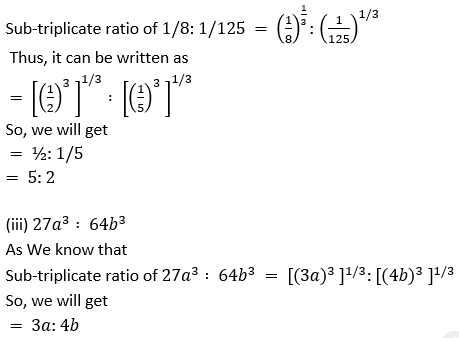7. Find out the reciprocal ratio of
(i) 4: 7
(ii) 32 ∶ 42
(iii) 1/9: 2
Solution:

(i) 4: 7
As We know that
Reciprocal ratio of 4: 7 = 7: 4

(ii) 32 ∶ 4^2
As We know that
Reciprocal ratio of 32 ∶ 42    = 42   ∶ 32  = 16: 9

(iii) 1/9: 2
As We know that
Reciprocal ratio of 1/9: 2 = 2: 1/9 = 18: 1

8. Arrange the following ratios in ascending order of magnitude:
2: 3,17: 21,11: 14 and 5: 7
Solution:
As It is given that
2: 3,17: 21,11: 14 and 5: 7

thus We can write it in fractions as
2/3,17/21,11/14,5/7
Here the LCM of 3,21,14 and 7 is 42

By converting the ratio as equivalent
2/3 = (2 × 14)/ (3 × 14) = 28/42
17/21 = (17 × 2)/ (21 × 2) = 34/ 42
11/14 = (11 × 3)/ (14 × 3) = 33/42
5/7 = (5 × 6)/ (7 × 6) = 30/42

Now writing it in ascending order
28/42,30/42,33/42,34/42

By further simplification
2/3,5/7,11/14,17/21

So we will get
2: 3,5: 7,11: 14 and 17: 21

9. (i) If A: B = 2: 3,B: C = 4: 5 and C: D = 6: 7,find A: D.
(ii) If x: y = 2: 3 and y: z = 4: 7,find x: y: z.
Solution:
(i) As we have given that
A: B = 2: 3,B: C = 4: 5 and C: D = 6: 7
We can write it as
A/ B = 2/3,B/C = 4/5,C/D = 6/7
By multiplication
A/B × B/C × C/D = 2/3 × 4/5 × 6/7
So we  will get
A/D = 16/35
A: D = 16: 35

(ii) as We know that the LCM of y terms 3 and 4 is 12
Now making equals of y as 12
x/y = 2/3 = (2 × 4)/ (3 × 4) = 8/12 = 8: 12
y/z = 4/7 × 3/3 = 12/21 = 12: 21
So x: y: z = 8: 12: 21

10. (i) If A: B = 1/4: 1/5 and B: C = 1/7: 1/6,find A: B: C.
(ii) If 3A = 4B = 6C,find A: B: C
Solution:
(i) As We know that
A: B = 1/4 × 5/1 = 5/4
B: C = 1/7 × 6/1 = 6/7
Here the LCM of B terms 4 and 6 is 12
Now making terms of B as 12

A/B = (5 × 3)/ (4 × 3) = 15/12 = 15: 12
B/C = (6 × 2)/ (7 × 2) = 12/14 = 12: 14
So A: B: C = 15: 12: 14

(ii) As we have given that
3A = 4B
Thus  We can write it as
A/B = 4/3
A: B = 4: 3
Similarly 4B = 6C
We can write it as
B/C = 6/4 = 3/2
B:C = 3: 2
So we will get
A: B: C = 4: 3: 2

11. (i) If 3x + 5y/ 3x – 5y = 7/3,find x: y.
(ii) If a: b = 3: 11,find (15a – 3b): (9a + 5b).
Solution:
(i) 3x + 5y/ 3x – 5y = 7/3
By cross multiplication
9x + 15y = 21x – 35y
By further simplification
21x – 9x = 15y + 35y
12x = 50y
So we will get
x/y = 50/12
= 25/6
Therefore, x: y = 25: 6

(ii) As we have given that
a: b = 3: 11
a/b = 3/11
It is given that
(15a – 3b)/ (9a + 5b)
Now dividing both numerator and denominator by b
= [15a/b – 3b/b]/ [9a/b + 5b/b]
By further calculation
= [15a/b – 3]/ [9a/b + 5]
Substituting the value of a/ b
= [15 × 3/11 – 3]/ [9 × 3/11 + 5]
So we will get
= [45/11 – 3]/ [27/11 + 5]
Taking LCM
= [(45 – 33)/ 11]/ [(27 + 55)/ 11]
= 12/11/ 82/11
thus We can write it as
= 12/11 × 11/82
= 12/82
= 6/41
Hence, (15a – 3b): (9a + 5b) = 6: 41.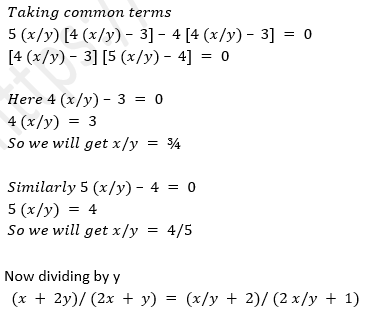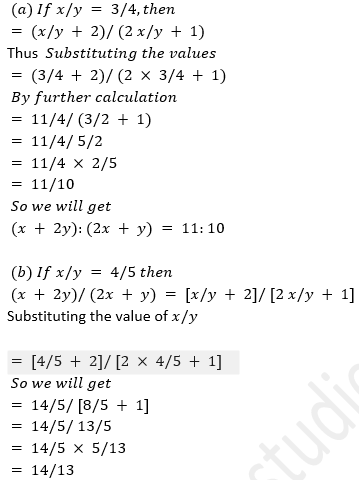We will get
(x + 2y)/ (2x + y) = 11/10 or 14/13
(x + 2y): (2x + y) = 11: 10 or 14: 13

(ii) y (3x – y): x (4x + y) = 5: 12
Thus It can be written asTaking common terms
5 (x/y) [4 (x/y) – 3] – 4 [4 (x/y) – 3] = 0
[4 (x/y) – 3] [5 (x/y) – 4] = 0

Here
4 (x/y) – 3 = 0
So we get
4 (x/y) = 3
x/y = 3/4

Similarly
5 (x/y) – 4 = 0
So we will get
5 (x/y) = 4
x/y = 4/5

(a) x/y = 3/4
We know that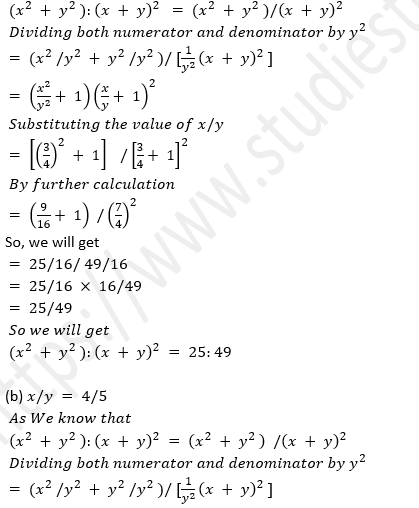13. (i) If (x – 9): (3x + 6) is the duplicate ratio of 4: 9, Find out the value of x.
(ii) If (3x + 1): (5x + 3) is the triplicate ratio of 3: 4, Find out the value of x.
(iii) If (x + 2y): (2x – y) is equal to the duplicate ratio of 3: 2, Find out x: y.
Solution: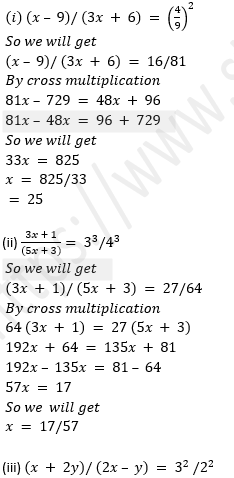So we will get
(x + 2y)/ (2x – y) = 9/4
By cross multiplication
9 (2x – y) = 4 (x + 2y)
18x – 9y = 4x + 8y
18x = 4x = 8y + 9y
So we get
14x = 17y
x/y = 17/14
x: y = 17: 14

14. (i) Find out two numbers in the ratio of 8: 7 such that when each is decreased by 12 ½, they are in the ratio 11: 9.
(ii) The income of a man is increased in the ratio of 10: 11. If the increase in his income is Rs 600 per month, Find out
Solution :

(i) Ratio = 8: 7
Lets assumed  the numbers as 8x and 7x
Using the condition
[8x – 25/2]/ [7x – 25/2] = 11/9
Taking LCM
[(16x – 25)/ 2]/ [(14x – 25)/ 2] = 11/9
By further calculation
[(16x – 25) × 2]/ [2 (14x – 25)] = 11/9
(16x – 25)/ (14x – 25) = 11/9
By cross multiplication
154x – 275 = 144x – 225
154x – 144x = 275 – 225
10x = 50
x = 50/10
= 5

So the numbers are
8x = 8 × 5 = 40
7x = 7 × 5 = 35

(ii) Lets assumed the present income = 10x
Increased income = 11x
So the increase per month = 11x – 10x = x
Here x = Rs 600
New income = 11x = 11 × 600 = Rs 6600

15. (i) A woman reduces her weight in the ratio 7: 5. What does her weight become if originally it was 91 kg.
(ii) A school collected Rs 2100 for charity. It was decided to divide the money between an orphanage and a blind school in the ratio of 3: 4. How much money did each receive?
Solution:
(i) Ratio of original and reduced weight of woman = 7: 5
Lets assumed original weight = 7x
Reduced weight = 5x
Here original weight = 91 kg
So the reduced weight = (91 × 5x)/ 7x = 65 kg

(ii) Amount collected for charity = Rs 2100
Here the ratio between orphanage and a blind school = 3: 4
Sum of ratios = 3 + 4 = 7

As We know that
Orphanage schools share = 2100 × 3/7 = Rs 900
Blind schools share = 2100 × 4/7 = Rs 1200

16. (i) The sides of a triangle are in the ratio 7: 5: 3 and its perimeter is 30 cm. Find out the lengths of sides.
(ii) If the angles of a triangle are in the ratio 2: 3: 4, Find out the angles.
Solution:
(i) As we have given that
Perimeter of triangle = 30 cm
Ratio among sides = 7: 5: 3
Here the sum of ratios = 7 + 5 + 3 = 15

As We know that
Length of first side = 30 × 7/15 = 14 cm
Length of second side = 30 × 5/15 = 10 cm
Length of third side = 30 × 3/15 = 6 cm

Therefore, the sides are 14 cm, 10 cm and 6 cm.

(ii) as We know that
Sum of all the angles of a triangle = 180°
Here the ratio among angles = 2: 3: 4
Sum of ratios = 2 + 3 + 4 = 917. Three numbers are in the ratio 1/2: 1/3: ¼. If the sum of their squares is 244, Find out the numbers.
Solution:
As we have given that
Ratio of three numbers = 1/2: 1/3: 1/4
= (6: 4: 3)/ 12
= 6: 4: 3

Lets assumed first number = 6x
Second number = 4x
Third number = 3x
So based on the condition
(6x)2  + (4x)2  + (3x)2  = 244
36x + 16x2  + 9x2   = 244
So we will get
61x = 244
x2  = 244/61 = 4 = 22
x = 2

Here
First number = 6x = 6 × 2 = 12
Second number = 4x = 4 × 2 = 8
Third number = 3x = 3 × 2 = 6

18. (i) A certain sum was divided among A, B and C in the ratio 7: 5: 4. If B got Rs 500 more than C, Find out the total sum divided.
(ii) In a business, A invests Rs 50000 for 6 months, B Rs 60000 for 4 months and C Rs 80000 for 5 months. If they together earn Rs 18800 Find out the share of each.
Solution:
(i) As we have given that
Ratio between A,B and C = 7: 5: 4
Consider A share = 7x
B share = 5x
C share = 4x
So the total sum = 7x + 5x + 4x = 16x

Based on the condition
5x – 4x = 500
x = 500
So the total sum = 16x = 16 × 500 = Rs 8000

(ii) 6 months investment of A = Rs 50000
1 month investment of A = 50000 × 6 = Rs 300000

4 months investment of B = Rs 60000
1 month investment of B = 60000 × 4 = Rs 240000

5 months investment of C = Rs 80000
1 month investment of C = 80000 × 5 = Rs 400000

Here the ratio between their investments = 300000: 240000: 400000 = 30: 24: 40
Sum of ratio = 30 = 24 + 40 = 94
Total earnings = Rs 18800

So we will get
A share = 30/94 × 18800 = Rs 6000
B share = 24/94 × 18800 = Rs 4800
C share = 40/94 = 18800 = Rs 8000